# Difference between revisions of "Factorial prime"

This article defines a property that can be evaluated for a prime number. In other words, every prime number either satisfies this property or does not satisfy this property.
View other properties of prime numbers | View other properties of natural numbers

## Definition

A factorial prime is a prime that differs from a factorial by. In other words, it is a prime of the form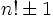.

## Occurrence

### Initial values

The initial values of factorial primes are given as: 2, 3, 5, 7, 23, 719, 5039, [SHOW MORE] View list on OEIS

The first four primes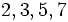are factorial primes. However, factorial primes become much rarer after that. The next two factorial primes are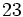and.

The initial values offor which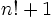is prime are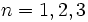. Note that, by Wilson's theorem,cannot be prime ifis prime, for. This explains, for instance, whyand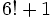are not prime.are also Brown numbers -- they are solutions to Brocard's problem ofbeing a perfect square.

The initial values offor which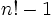is prime are: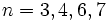.